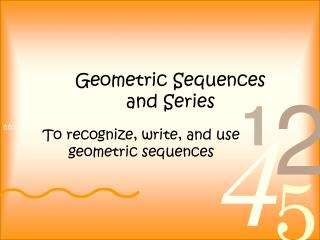Download PresentationGeometric Sequences and Series

# Geometric Sequences and Series - PowerPoint PPT Presentation

Geometric Sequences and Series. To recognize, write, and use geometric sequences. Geometric Sequence. A geometric sequence is a sequence that satisfies a n = a n-1 r where r is the common ratio. The common ratio r = or . Example 1:.I am the owner, or an agent authorized to act on behalf of the owner, of the copyrighted work described.
Download Presentation## Geometric Sequences and Series

Download Policy: Content on the Website is provided to you AS IS for your information and personal use and may not be sold / licensed / shared on other websites without getting consent from its author.While downloading, if for some reason you are not able to download a presentation, the publisher may have deleted the file from their server.

- - - - - - - - - - - - - - - - - - - - - - - - - - E N D - - - - - - - - - - - - - - - - - - - - - - - - - -
Presentation Transcript
1. Geometric Sequences and Series To recognize, write, and use geometric sequences

2. Geometric Sequence • A geometric sequence is a sequence that satisfies an = an-1r where r is the common ratio. • The common ratio r = or .

3. Example 1: • Determine whether or not the following sequence is geometric. If it is, find the common ratio. 60, 30, 0,  30,  60, . . . There is not a constant ratio, so it isn’t a geometric sequence.

4. Example 1b: • Find the common ratio of the following geometric sequences. 3, 6, 12, 24, … r = 2 1, ½, ¼, ⅛, … r = ½

5. The nth Term a1 = a1 a2 = a1r a3 = a2r = a1r·r = a1r2 a4 = a3r = a1r3 a5 = a4r= a1r4 an = a1rn-1

6. Geometric Sequences • Every geometric sequence can be written in the form a1, a1r, a1r2, a1r3, … a1rn-1 • A geometric sequence may be thought of as an exponential function whose domain is the set of natural numbers.

7. Example 2: • Write the first five terms of the geometric sequence whose first term is a1 = 5 and whose common ratio is - 3. 5, -15, 45, -135, 405

8. Example 3: • Find the eighteenth term of the geometric sequence that begins with 15, 12, 9.6, 7.68 . . . . 1 1 a18 = .3378